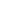#SIX-3013 Probability Distributions is a Course

## SIX-3013 Probability Distributions

Self-paced

### Full course description

Course Description

A random experiment or sample can result in different outcomes. The probability that each outcome will occur can be calculated and charted using different probability distributions.

By the end of this course, you will be able to

• Describe and interpret normal, binomial, Poisson, t, chi square, and F distributions

• Identify formulas for calculating the probability of data values of different probability distributions

Estimated completion time (hours): 2.2